Courses

# Solutions of Magnetic Effects of Electric Current (Page No- 84) - Physics By Lakhmir Singh, Class 10 Class 10 Notes | EduRev

## Class 10 : Solutions of Magnetic Effects of Electric Current (Page No- 84) - Physics By Lakhmir Singh, Class 10 Class 10 Notes | EduRev

The document Solutions of Magnetic Effects of Electric Current (Page No- 84) - Physics By Lakhmir Singh, Class 10 Class 10 Notes | EduRev is a part of Class 10 category.
All you need of Class 10 at this link: Class 10

Lakhmir Singh Physics Class 10 Solutions Page No:84

Question 37: In the straight wire A, current is flowing in the vertically downward direction whereas in wire B the current is flowing in the vertically upward direction. What is the direction of magnetic field :
(a) in wire A ?
(b) in wire B ?
Name the rule which you have used to get the answer.

Solution :

(a) Clockwise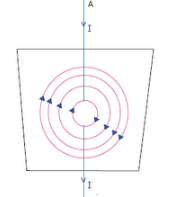(b) Anticlockwise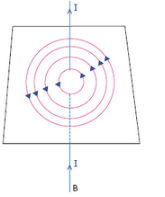We have used Maxwell's right hand thumb rule here

Question 38: The figure shows a solenoid wound on a core of soft iron. Will the end A be a N pole or S pole when the current flows in the direction shown ?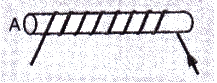Solution : End A will be a S-pole because current flows in the clockwise direction at A.

Question 39: A current-carrying straight wire is held in exactly vertical position. If the current passes through this wire in the vertically upward direction, what is the direction of magnetic field produced by it ? Name the rule used to find out the direction of magnetic field.

Solution : Direction of magnetic field is anticlockwise.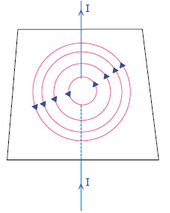Maxwell’s right hand thumb rule. is used to find out the direction of magnetic field.

Question 40: For the coil in the diagram below, when the switch is pressed :
(a) what is the polarity of end A ?
(b) which way will the compass point then ?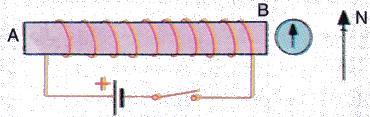Solution : (a) End A becomes S-pole because current flows in clockwise direction at A.
(b) When A becomes S-pole, the other end becomes N-pole. So the tip of the compass (with also has North polarity) moves away from this end i.e., tip moves towards right.

Question 41: A current flows downwards in a wire that passes vertically through a table top. Will the magnetic field lines around it go clockwise or anticlockwise when viewed from above the table ?

Solution : Magnetic field lines around it will be clockwise (according to Maxwell’s right hand thumb rule).

Question 42: The directions of current flowing in the coil of an electromagnet at its two ends X and Y are as shown below:
(a) What is the polarity of end X ?
(b) What is the polarity of end Y ?
(c) Name and state the rule which you have used to determine the polarities.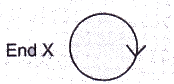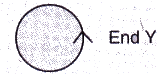Solution : (a) End X is S-pole (because current flows in clockwise direction).
(b) End Y is N-pole (because current flows in anticlockwise direction).
(c) Clock face rule – Looking at the face of a loop, if the current around that face is in anticlockwise direction, the face has north polarity, while if the current at that face is in clockwise direction, the face has south polarity.

Question 43: The magnetic field associated with a current-carrying straight conductor is in anticlockwise direction. If the conductor was held along the east-west direction, what will be the direction of current through it ? Name and state the rule applied to determine the direction of current ?

Solution : The direction of current will be from east to west.
We have applied MAxwell’s right hand thumb rule here.
According to Maxwell’s right hand thumb rule: Imagine that you are grasping the current-carrying wire in your right hand so that your thumb points in the direction of current, then the direction in which your fingers encircle the wire will give the direction of magnetic field lines around the wire.

Question 44: A current-carrying conductor is held in exactly vertical direction. In order to produce a clockwise magnetic field around the conductor, the current should be passed in the conductor :
(a) from top towards bottom
(b) from left towards right
(c) from bottom towards top
(d) from right towards left

Solution : (a) from top towards bottom.

Question 45: A thick wire is hanging from a wooden table. An anticlockwise magnetic field is to be produced around the wire by passing current through this wire by using a battery. Which terminal of the battery should be connected to the :
(a) top end of wire ?
(b) bottom end of wire ?
(c) Give reason for your choice.

Solution : (a) Negative terminal
(b) Positive terminal
Because the current should be passed into wire upwards.

Offer running on EduRev: Apply code STAYHOME200 to get INR 200 off on our premium plan EduRev Infinity!

,

,

,

,

,

,

,

,

,

,

,

,

,

,

,

,

,

,

,

,

,

,

,

,

;